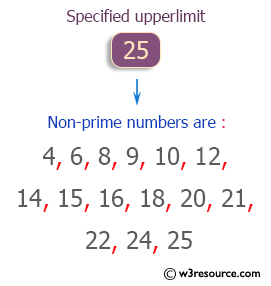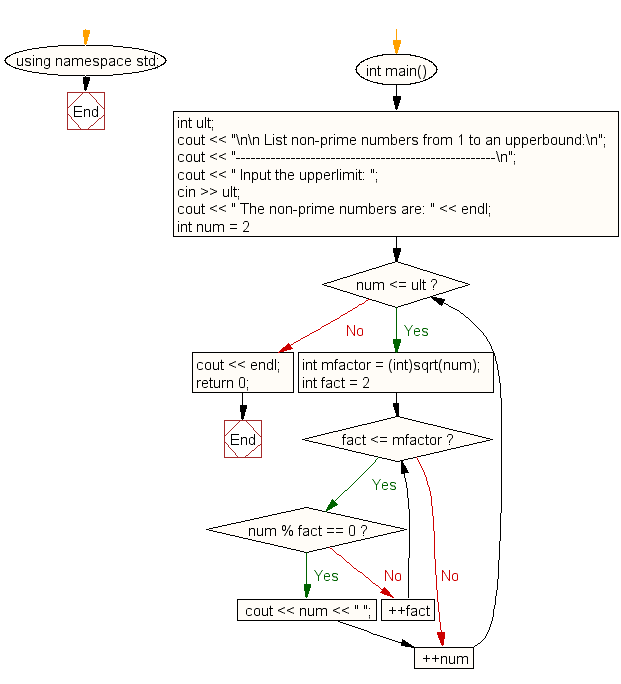﻿ C++ Exercises: List non-prime numbers from 1 to an upperbound - w3resource

# C++ Exercises: List non-prime numbers from 1 to an upperbound

## C++ For Loop: Exercise-16 with Solution

Write a C++ program to list non-prime numbers from 1 to an upperbound.

Pictorial Presentation:Sample Solution :-

C++ Code :

``````#include <iostream>
#include <cmath>
using namespace std;

int main()
{
int ult;
cout << "\n\n List non-prime numbers from 1 to an upperbound:\n";
cout << "----------------------------------------------------\n";
cout << " Input the upperlimit: ";
cin >> ult;
cout << " The non-prime numbers are: " << endl;
for (int num = 2; num <= ult; ++num)
{
int mfactor = (int)sqrt(num);
for (int fact = 2; fact <= mfactor; ++fact)
{
if (num % fact == 0)
{
cout << num << " ";
break;
}
}
}
cout << endl;
return 0;
}
``````

Sample Output:

``` List non-prime numbers from 1 to an upperbound:
----------------------------------------------------
Input the upperlimit: 25
The non-prime numbers are:
4 6 8 9 10 12 14 15 16 18 20 21 22 24 25
```

Flowchart:C++ Code Editor: